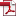• KSII Transactions on Internet and Information Systems
Monthly Online Journal (eISSN: 1976-7277)

# Secure Outsourced Computation of Multiple Matrix Multiplication Based on Fully Homomorphic Encryption

Vol. 13, No. 11, November 30, 201910.3837/tiis.2019.11.019, Download Paper (Free):#### Abstract

Fully homomorphic encryption allows a third-party to perform arbitrary computation over encrypted data and is especially suitable for secure outsourced computation. This paper investigates secure outsourced computation of multiple matrix multiplication based on fully homomorphic encryption. Our work significantly improves the latest Mishra et al.셲 work.We improve Mishra et al.셲 matrix encoding method by introducing a column-order matrix encoding method which requires smaller parameter. This enables us to develop a binary multiplication method for multiple matrix multiplication, which multiplies pairwise two adjacent matrices in the tree structure instead of Mishra et al.셲 sequential matrix multiplication from left to right. The binary multiplication method results in a logarithmic-depth circuit, thus is much more efficient than the sequential matrix multiplication method with linear-depth circuit. Experimental results show that for the product of ten 32횞32 (64횞64) square matrices our method takes only several thousand seconds while Mishra et al.셲 method will take about tens of thousands of years which is astonishingly impractical. In addition, we further generalize our result from square matrix to non-square matrix. Experimental results show that the binary multiplication method and the classical dynamic programming method have a similar performance for ten non-square matrices multiplication.